## Vectors A, B, C and D have the following components: X components (A, B, C and D respectively): +2.5 units, +6.1 units, -3.6 units and

Question

Vectors A, B, C and D have the following components:
X components (A, B, C and D respectively): +2.5 units, +6.1 units, -3.6 units and -1.5 units
Y components (A, B, C and D respectively): +4.3 units, -2.1 units, +1.0 units and -7.3 units

The magnitude of the resultant is:________

in progress 0
6 months 2021-07-27T10:54:19+00:00 1 Answers 4 views 0

The magnitude of the resultant is approximately 5.391 units.

Explanation:

We notice that vectors have the following characteristics: A magnitude and direction. That is: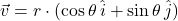Where: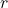– Magnitude, dimensionless.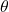– Direction, measured in radians.

This expression can be rearranged as: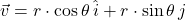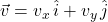Where: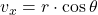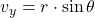If we know a set of vectors of the form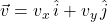, the resultant vector (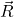) is the vectorial sum of every vector in the set: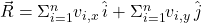If we know that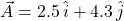,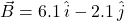,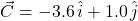and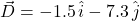, the resultant vector is: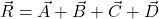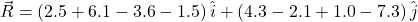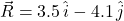And lastly, we obtain the magnitude of the resultant by Pythagoras’ Theorem: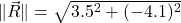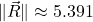The magnitude of the resultant is approximately 5.391 units.